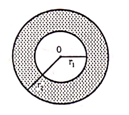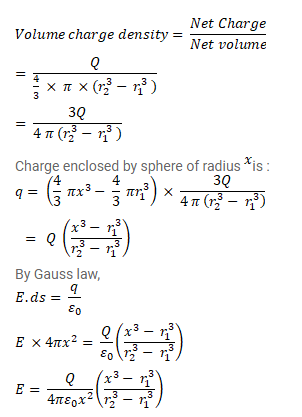# A charge Q is distributed uniformly within the material ofQuestion:

A charge $\mathrm{Q}$ is distributed uniformly within the material of a hollow sphere of inner and outer radii $r_{1}$ and $r_{2}{ }^{\circ}$ Find the electric field at a point $\mathrm{P}$ a distance ${ }^{x}$ away from the centre for $\mathrm{r}_{1}<\mathrm{x}<\mathrm{r}_{2}$ Draw a rough graph showing the electric field as a function of $x$ for $0<\mathrm{x}<2 \mathrm{r}_{2}$.Solution: